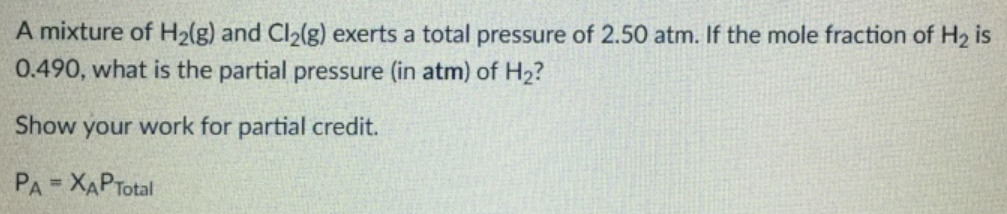Chemistry Practice Problems Partial Pressure Practice Problems Solution: A mixture of H2(g) and Cl2(g) exerts a total press...

🤓 Based on our data, we think this question is relevant for Professor Delgado's class at FIU.

# Solution: A mixture of H2(g) and Cl2(g) exerts a total pressure of 2.50 atm. If the mole fraction of H2 is 0.490, what is the partial pressure (in atm) of H2? Show your work for partial credit. PA = XAPTotal

###### Problem

A mixture of H2(g) and Cl2(g) exerts a total pressure of 2.50 atm. If the mole fraction of H2 is 0.490, what is the partial pressure (in atm) of H2? Show your work for partial credit. P= XAPTotalView Complete Written Solution

Partial Pressure

Partial Pressure

#### Q. Part A: What is the mole fraction of oxygen gas in air (see table 5.3 in the textbook)? Express your answer using two significant figures. Part B: Wha...

Solved • Sun May 27 2018 12:48:18 GMT-0400 (EDT)

Partial Pressure

#### Q. A sample of 5.10 mL of diethylether (C2H5OC2H5; density = 0.7134 g/mL) is introduced into a 6.50 -L vessel that already contains a mixture of N2 and O...

Solved • Sun May 27 2018 10:22:10 GMT-0400 (EDT)

Partial Pressure

#### Q. A mixture of 0.164 moles of C is reacted with 0.117 moles of O2 in a sealed, 10.0 L vessel at 500 K, producing a mixture of CO and CO2. [See reaction ...

Solved • Mon May 21 2018 03:00:39 GMT-0400 (EDT)

Partial Pressure

#### Q. A mixture of 0.220 moles CO, 0.350 moles H 2 and 0.640 moles He has a total pressure of 2.95 atm. What is the pressure of H2 (in atm)?a. 1.56 atmb. 0....

Solved • Fri Mar 23 2018 15:21:13 GMT-0400 (EDT)